Numerical Solutions to ODEs

Mathematica cannot find a direct solution to the following nonlinear ODE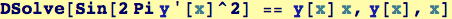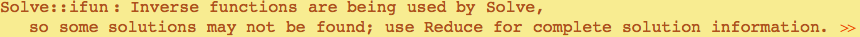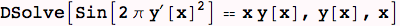NDSolve is a numerical method for finding a solution. An initial condition and the desired range of solution are required.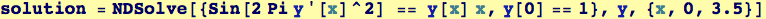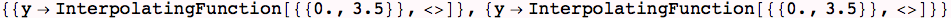The results look kind of strange, perhaps, but they are a set of rules that provide a function that interpolates between values.
Here is how to find the approximate solution at three different values of x on the specified interval: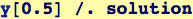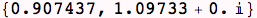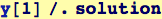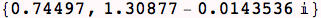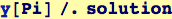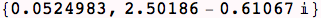Created by Wolfram Mathematica 6.0  (10 November 2007)